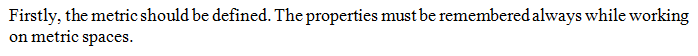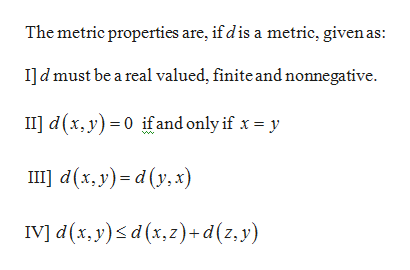# Real AnalysisWe started metric spaces yesterday.  I am asked to prove that the metric space defined as P<x1,y1> and Q<x2,y2> is a metric space.  When I look at examples of these proofs they all say that the proof of the first properties (positive definiteness and symmetry) are trivial.  I think I must be more complete than that.  How do I best show that these properties are true for this space? Further, how do I show that the triangle inequality is true?   Do I create a new ordered pair and call it R<x3,y3> and then prove that the distance between P and Q is less than or equal to the distances from P to R + R to Q?

Question

Real Analysis

We started metric spaces yesterday.  I am asked to prove that the metric space defined as P<x1,y1> and Q<x2,y2> is a metric space.  When I look at examples of these proofs they all say that the proof of the first properties (positive definiteness and symmetry) are trivial.  I think I must be more complete than that.  How do I best show that these properties are true for this space?

Further, how do I show that the triangle inequality is true?   Do I create a new ordered pair and call it R<x3,y3> and then prove that the distance between P and Q is less than or equal to the distances from P to R + R to Q?

check_circle

Step 1...help_outlineImage TranscriptioncloseThe metric properties are, if dis a metric, given as: Ild must be a real valued, finite and nonnegative. II d(x, y)0 if and only if x y d(x, y)d (y,x) IV] d(x, y)d(x,z)+ d(z,y) fullscreen

### Want to see the full answer?

See Solution

#### Want to see this answer and more?

Solutions are written by subject experts who are available 24/7. Questions are typically answered within 1 hour.*

See Solution
*Response times may vary by subject and question.
Tagged in

### Math